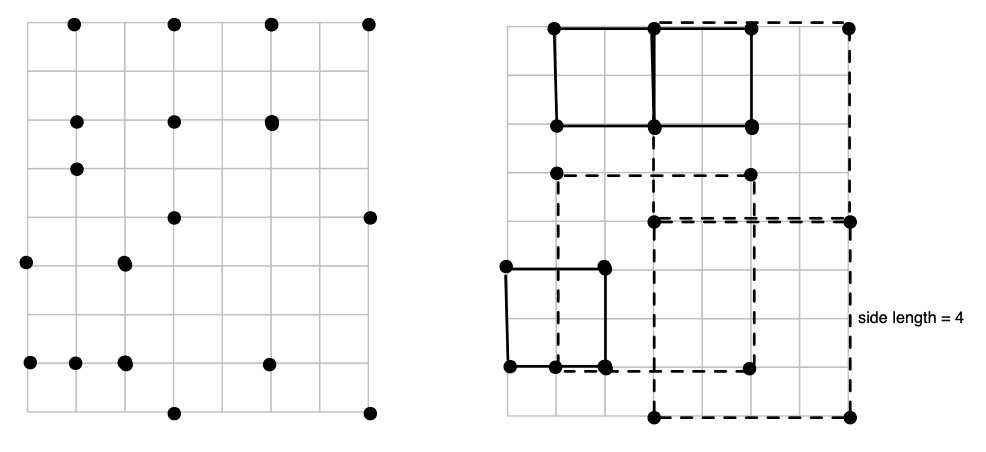시간 제한 메모리 제한 제출 정답 맞은 사람 정답 비율
1 초 128 MB 1 1 1 100.000%

## 문제

As Sam is flying over the Grid world to the outlands, he notices some interesting formations on the ground, where there is well-defined small square grid with only a subset of its grid points lit (see figure). To pass time, Sam starts counting the number of different axis-parallel squares formed by the grid points. You are to write a program to help Sam with this task.Specifically, given a set of grid coordinates, your goal is to find the most common size of an axis-parallel square formed by subsets of the given points. An axis-parallel square is such that the square is aligned with the grid axes (i.e., the sides of the square are either vertical or horizontal, but are not allowed to at an angle). For instance, in the figure above, there are 3 such squares with side length 2, and three squares with side length 4.

## 입력

The first line in the test data file contains the number of test cases (≤ 50). After that, each line contains one test case. The test case begins with the number of points, k (≤ 30), and then we have k coordinate pairs (for a total of 2k numbers). Assume all numbers are ≥ 0, and that the coordinates are all ≤ 30.

## 출력

For each test case, if there is at least one axis-parallel square present among the provided points, you should output the side length for which there are maximum squares present, and also the number of squares for that side length. If there are more than two side lengths with maximum number of squares, then output the largest of the side lengths. If the provided set of points does not form a single square, then you should output: No squares among the points.

## 예제 입력 1

8
4 0 0 1 1 0 1 1 0
4 0 0 2 1 0 1 1 0
4 0 0 2 2 0 2 2 0
9 0 0 0 1 0 2 1 0 1 1 1 2 2 0 2 1 2 2
9 0 0 0 2 0 4 2 0 2 2 2 4 4 0 4 2 4 4
10 2 3 0 2 2 1 2 0 1 1 1 2 3 3 3 0 0 1 0 0
10 2 0 3 2 0 1 2 1 1 0 3 3 1 3 2 3 2 2 3 0
10 3 1 2 2 3 3 0 2 0 0 2 0 0 1 1 1 1 3 1 2


## 예제 출력 1

LENGTH = 1, COUNT = 1
No squares among the points.
LENGTH = 2, COUNT = 1
LENGTH = 1, COUNT = 4
LENGTH = 2, COUNT = 4
LENGTH = 1, COUNT = 1
LENGTH = 1, COUNT = 1
LENGTH = 2, COUNT = 2